Courses

# Test: Digital Filters Design Consideration

## 10 Questions MCQ Test Digital Signal Processing | Test: Digital Filters Design Consideration

Description
This mock test of Test: Digital Filters Design Consideration for Electrical Engineering (EE) helps you for every Electrical Engineering (EE) entrance exam. This contains 10 Multiple Choice Questions for Electrical Engineering (EE) Test: Digital Filters Design Consideration (mcq) to study with solutions a complete question bank. The solved questions answers in this Test: Digital Filters Design Consideration quiz give you a good mix of easy questions and tough questions. Electrical Engineering (EE) students definitely take this Test: Digital Filters Design Consideration exercise for a better result in the exam. You can find other Test: Digital Filters Design Consideration extra questions, long questions & short questions for Electrical Engineering (EE) on EduRev as well by searching above.
QUESTION: 1

### The ideal low pass filter cannot be realized in practice.

Solution:

Explanation: We know that the ideal low pass filter is non-causal. Hence, a ideal low pass filter cannot be realized in practice.

QUESTION: 2

### The following diagram represents the unit sample response of which of the following filters?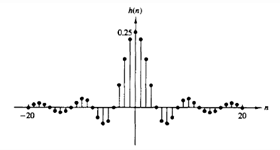Solution:

Explanation: At n=0, the equation for ideal low pass filter is given as h(n)=ω/π.
From the given figure, h(0)=0.25=>ω=π/4.
Thus the given figure represents the unit sample response of an ideal low pass filter at ω=π/4.

QUESTION: 3

### If h(n) has finite energy and h(n)=0 for n<0, then which of the following are true?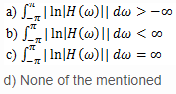Solution:

Explanation: If h(n) has finite energy and h(n)=0 for n<0, then according to the Paley-Wiener theorem, we have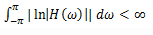QUESTION: 4

If |H(ω)| is square integrable and if the integral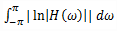is finite, then the filter with the frequency response

Solution:

Explanation: If |H(ω)| is square integrable and if the integral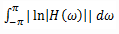is finite, then we can associate with |H(ω)| and a phase response θ(ω), so that the resulting filter with the frequency response H(ω)=|H(ω)|ejθ(ω) is causal.

QUESTION: 5

The magnitude function |H(ω)| can be zero at some frequencies, but it cannot be zero over any finite band of frequencies.

Solution:

Explanation: One important conclusion that we made from the Paley-Wiener theorem is that the magnitude function |H(ω)| can be zero at some frequencies, but it cannot be zero over any finite band of frequencies, since the integral then becomes infinite. Consequently, any ideal filter is non-causal.

QUESTION: 6

If h(n) is causal and h(n)=he(n)+ho(n),then what is the expression for h(n) in terms of only he(n)?

Solution:

Explanation: Given h(n) is causal and h(n)= he(n)+ho(n)
=>he(n)=1/2[h(n)+h(-n)] Now, if h(n) is causal, it is possible to recover h(n) from its even part he(n) for 0≤n≤∞ or from its odd component ho(n) for 1≤n≤∞.
=>h(n)= 2he(n)u(n)-he(0)δ(n) ,n ≥ 0.

QUESTION: 7

If h(n) is causal and h(n)=he(n)+ho(n),then what is the expression for h(n) in terms of only ho(n)?

Solution:

Explanation: Given h(n) is causal and h(n)= he(n)+ho(n)
=>he(n)=1/2[h(n)+h(-n)] Now, if h(n) is causal, it is possible to recover h(n) from its even part he(n) for 0≤n≤∞ or from its odd component ho(n) for 1≤n≤∞.
=>h(n)= 2ho(n)u(n)+h(0)δ(n) ,n ≥ 1
since ho(n)=0 for n=0, we cannot recover h(0) from ho(n) and hence we must also know h(0).

QUESTION: 8

If h(n) is absolutely summable, i.e., BIBO stable, then the equation for the frequency response H(ω) is given as?

Solution:

Explanation: . If h(n) is absolutely summable, i.e., BIBO stable, then the frequency response H(ω) exists and
H(ω)= HR(ω)+j HI(ω)
where HR(ω) and HI(ω) are the Fourier transforms of he(n) and ho(n) respectively.

QUESTION: 9

HR(ω) and HI(ω) are interdependent and cannot be specified independently when the system is causal.

Solution:

Explanation: Since h(n) is completely specified by he(n), it follows that H(ω) is completely determined if we know HR(ω). Alternatively, H(ω) is completely determined from HI(ω) and h(0). In short, HR(ω) and HI(ω) are interdependent and cannot be specified independently when the system is causal.

QUESTION: 10

The frequency ωP is called as:

Solution:

Explanation: Pass band edge ripple is the frequency at which the pass band starts transiting to the stop band.